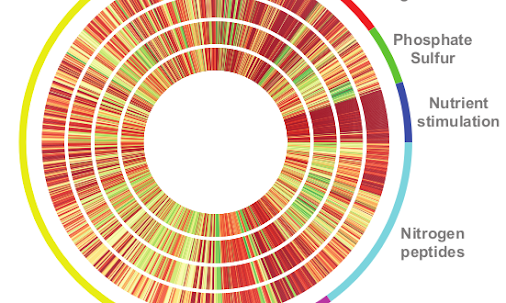Matplotlib: draw a series of radial lines on PolarAxes
I’m trying to replicate a certain plot using matplotlib: it should look something like this. I have seen that it it possible to use the PolarAxes to draw radial points: for istance i’ve made a really simple polar plot with the following snippet: import matplotlib.pyplot as plt fig = plt.figure() # Set the axes as polar ax = fig.add_subplot(111, polar=True) # Draw some points ax.plot(,, ‘o’) ax.plot(,, ‘o’) ax.plot(,, ‘o’) # Go …

Post imported by Google+Blog for WordPress.